# ICSE Solutions for Chapter 12 Radioactivity Class 10 Selina Physics

### Exercise 12 A

Question 1: What do you understand by the term radioactivity?

Answer 1: The process of self, spontaneous and random emission of a or P and y radiations from the nucleus of elements of atomic number higher than 82, is called radioactivity.

Question 2: What are radioactive substances? Give two examples of such substances.

Answer 2: Radioactive substances are those which have the property of self emission of α or β and γ radiations.
Examples: Uranium, Thorium.

Question 3: Name the different radiations which are emitted by the radioactive substances.
Are all the radiations mentioned by you, emitted in a single radioactive decay?

(i) Alpha (α), (ii) Beta (β), and (iii) Gamma (γ).
All the above radiations are not emitted in a single radioactive decay. There will be either an α emission or a β emission, which may be accompained by γ-emission.

Question 4: Are all the radiations viz. α, β and γ emitted in a single radioactive decay?

Answer 4: All the above radiations are not emitted in a single decay. There will be either an α emission or a β emission, which may be accompanied by γ-emission.

Question 5: Compare the penetrating powers of α, β and γ-radiations.

Question 6: Compare the ionising powers of α, β and γ  radiations.

Question 7: State the kind of nuclear reaction taking place in a nucleus during the emission of a β-particle.

Answer 7: In the process of emission of a β-particle from the nucleus, a neutron changes into a proton by emitting a β-particle (electron).Hence in the nucleus, the number of neutrons decreases by 1 with the emission of a β-particle and the number of protons increases by one. So the sum of protons and neutrons, i.e., atomic mass remains the same but the atomic number increases by one.

Question 8: A certain nucleus has a mass number 20 and atomic number 9. Find the number of neutrons and protons present in it.

Answer 8: (i) Number of neutrons = Mass number – Atomic number
= 20 – 9 = 11
(ii) Number of protons = Atomic number = 9.

Question 9: Justify with reasons, whether the following nuclear reactions are allowed or not.

Answer 9: (i) This reaction is allowed because, the mass number as well as atomic number are conserved here.
(ii) This reaction is not allowed because even though charge (atomic number) is conserved in it, the mass number [= A on the L H.S. and equal to (A + 4)] on the R.H.S. is not conserved.

Question 10: An electrons emitter must have how much work function and melting point.

Answer 10: An electron emitter must have low work function and high melting point.

Question 11: A radioactive nucleus undergoes a series of decays according to the sequence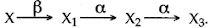If the mass number and atomic number of X3 are 172 and 69 respectively, what is the mass number and atomic number of X?

Answer 11: Mass no. of X = 180
Atomic no. of X = 72

Question 12: A mixture of radioactive substances gives off three types of radiations:
(i) Name the radiation which travels with the speed of light.
(ii) Name the radiation which has the highest ionizing power.

(ii) α-particles.

Question 13: Complete the following nuclear changes:Question 14: An imaginary radioactive element 92X235 decays to form X1, X2, X3, X4, X5 and X6 nuclides, by ejecting two β-particles, followed by α-particles and again two β-particles, followed by α-particle. Represent the various nuclear change in the form of equation. State the mass number and atomic number of X6. List the isotopes and isobars in the above nuclear equation.

The mass number and atomic number of X6 is 227 and 92 respectively.

Isotopes: (a) 92X234, 92X3231, 92X6227 (b) 93X1235, 93X4231 (c) 94X2235, 94X5231

Isobars: (a) 92X235, 93X1235, 94X2235 (b) 92X3231, 93X4231, 94X5231

Question 15: State two factors on which the rate of emission of thermions depends.

Answer 15: Rate of emission of thermions depends on:
(i) The material of the surface
(ii) The temperature of the surface.

Question 16: The isotope of 92U235  decays by alpha emission to an isotope of Thorium (Th). The Thorium isotope decays by beta emission to an isotope of Protactinium (Pa). Write down the equations to represent these two nuclear changes.Question 17: A mixture of radioactive substances gives off three types of radiations.
(i) Name the radiations which are charged.
(ii) Name the radiation similar in nature to cathode rays.
(iii) Name the radiation similar in nature to X-rays.
(iv) Name the radiation which is deviated most in a magnetic field.
(v) Name the radiation which is not affected by an electric field.
(vi) Name the radiation which has the maximum penetrating power.
(vii) Name the radiation which has the highest ionising power.
(viii) Name the radiation which has the highest speed.
(ix) Name the radiation which when becomes neutral, become the atom of a rare gas.

(ii) Beta radiation is similar in nature to cathode rays.
(iii) Gamma radiation is similar in nature to X-rays.
(iv) Beta radiation deviates most in a magnetic field.
(v) Gamma radiation is not affected by an electric field.
(vi) Gamma radiation has the maximum penetrating power.
(vii) Alpha radiation has the highest ionising power.
(viii) Gamma radiation has the highest speed.
(ix) Alpha radiation when become neutral become the atom of rare (helium) gas.

Question 18: State, giving reasons, whether the following nuclear decays are allowed or not?

Answer 18: (i) This nuclear decay is allowed since the atomic number and mass number are conserved.
(ii) This nuclear decay is not allowed since the mass number is not conserved.
(iii) This nuclear decay is not allowed since the atomic number and mass number both are not conserved.

Question 19: The nucleus  84X202 emits an alpha particle and forms the nucleus Y. Represent this change in the form of an equation.Question 20: What changes will take place in the mass number and atomic number of the nucleus Y if it emits gamma radiations?

Answer 20: No change in mass number and atomic number when γ radiations are emitted out.

Question 21: How does the position of an element change in the periodic table when it emits out an alpha particle?

Answer 21: When an element emits out an alpha particle, a new element is formed with properties similar to those of an element two places earlier in the periodic table.

Question 22: What changes occur in the nucleus of a radioactive element when it emits an alpha particle? Show by an example?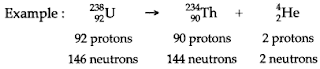Question 23: What will an alpha particle change into when it absorbs:
(i) One electron; (ii) Two electrons?

Answer 23: (i) Single ionised Helium (He+), (ii) Helium atom.

Question 24: State three properties of α-rays.

Answer 24: (i) Alpha rays are composed of helium nuclei.
(ii) Alpha rays ionise the gas through which they pass.
(iii) Alpha rays are deflected by the electric and magnetic fields.

Question 25: What is the effect on the atomic mass and the atomic number of a radioactive element, after the emission of an α-particle?

Answer 25: During the emission of an α-particle from the nucleus of a radioactive element, its atomic mass decreases by 4 and the atomic number also decreases by 2. If ZXA represents a radioactive element having atomic mass A and atomic number Z, then:Question 26: When an alpha particle gains two electrons it becomes neutral and becomes an atom of an element which is a rare gas. What is the name of this rare gas?

Question 27: An atomic nucleus A is composed of 84 protons and 128 neutrons. It emits an α-particle and is transformed into nucleus B. Write down the composition of B.

Answer 27: A emits an α-particle, so in the nucleus of B, neutrons will be 128 – 2 = 126 and protons will be 84 – 2 = 82.

Question 28: State charge and mass of (i) α-particle, (ii) β-particle.

Answer 28: (i) The charge on α-particle is 3.2 ×10-19 coulomb and its mass is 6.67 × 10-27 kg (i.e., nearly 4 times the mass of proton).
(ii) The charge on β-particle is – 1.6 × 10-19 coulomb and its mass is 9.1 × 10-31 kg.

Question 29: How does the position of an element change in the periodic table when it emits out a beta particle?

Answer 29: When an element emits out a beta particle, a new element is formed with properties similar to those of an element one place later in the periodic table.

Question 30: What are α-and β-radiations?

Answer 30: α radiations are positively charged particles with charge + 2e and mass 4mp where e is electronic charge (i.e., charge on a proton) and mp is the mass of a proton.
β radiations are negatively charged particles (i.e., electrons) with charge – e and mass 1/1836 times the mass of a proton.
γ-radiation are electromagnetic waves of wavelength in the range 0.001 Å.

Question 31: State three common properties of beta rays and cathode rays.

Answer 31: (i) Beta rays and cathode rays both are composed of electrons.
(ii) Beta rays and cathode rays both are deflected by the electric and magnetic fields in the same direction.
(iii) Beta rays and cathode rays both ionise the gas through which they pass.

Question 32: The nucleus of an atom does not contain electrons. Explain how is it possible for the nucleus to emit a beta particle which is fast moving electron.

Answer 32: The nucleus emits a beta particle when a neutron inside it changes into a proton. The reaction isQuestion 33: What happens to the atomic number of an element when it emits:
(i) An alpha particle; (ii) A beta particle.Question 34: A radioactive carbon nucleus 6C14 emits a β-particle to form a nitrogen nucleus. Represent the change by an equation showing the atomic and mass number of each elements.

Answer 34: When the nucleus of an element emits a beta particle, the number of protons increases by 1 and number of neutrons decreases by 1.Question 35: Two radioactive nuclei are represented by xAp and yAand when p and q are mass numbers and x, y are the atomic number. How can the products be represented, i .e., what are the new values of p, q. x and y after the emission of an α-particle and a β-particle from A and B respectively.Question 36: A nucleus of an element has the symbol 84P202, and emits an α-particle and then a β-particle. The final nucleus is bQa Find a and b.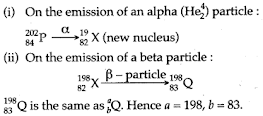Question 37: The nucleus B emit a β-particle and is transformed into nucleus C. What is the composition of C?

Answer 37: With the emission of a β-particle from nucleus B, number of protons will increase by one, i.e., number of protons = 82 + 1 = 83 and number of neutrons will be 126 – 1 = 125, i.e., the nucleus C will have 83 protons and 125 neutrons.
Mass number of nucleus A = Number of protons + number of neutrons
= 84 + 128 = 212.

Question 38: Explain briefly what changes take place within the nuclei when beta particles are emitted by a radioactive substance.

Answer 38: The mass number remains unchanged but the atomic number increases by 1 of the nuclei undergoing such a change.

Question 39: Which part of the atom undergoes a change in the process of radioactive decay?

Answer 39: The nucleus of the atom undergoes a change in the process of radioactive decay.

Question 40: How do infrared and γ-rays differ in (i) wavelength, (ii) penetrating power?

Answer 40: (i) Infrared rays are of much longer wavelength than that of γ rays. The wavelength of infrared rays lies between 8000 Å to 107 Å while that of γ rays lies between 0.001 Å to 0.1 Å.
(ii) γ rays are most penetrating while infrared rays almost have a negligible penetrating power.

Question 41: From α, β and γ-rays, name the one which travels with the speed of light?

Answer 41: γ-rays travel with the speed of light.

Question 42: From α, β and γ-rays, name the one which is not affected by an electric or magnetic field?

Answer 42: γ-rays are not affected by electric or magnetic field.

Question 43: Arrange α, β and γ-rays in ascending order with respect to their (i) Penetrating power,
(ii) Ionizing power and (iii) Biological effect.

Question 44: What changes occur in the nucleus of radioactive element when it emits gamma radiation? Give an example.

Answer 44: When the nucleus of a radioactive element emits gamma radiation, there is no change inside the nucleus except that its excess energy is released in the form of y-radiation.The star indicates the excited state of nucleus.

Question 45: What is the value of the speed of gamma radiations in air or vacuum?

Answer 45: Speed of γ radiation = 3×108 m/s in air or vacuum.

Question 46: What are beta rays and gamma rays?

Answer 46: β-rays are a stream of electrons emitted by the nucleus of an atom and γ-rays are electromagnetic waves of high frequency given off by nuclei.

Question 47: Name the radioactive radiations which have the least penetrating power.

Question 48: Explain why alpha and beta-particles are deflected in an electric or a magnetic field but gamma: rays are not deflected in such a field.

Answer 48: α and β are positive and negative charged particles respectively, therefore these are deflected in electric or magnetic field whereas γ radiations are not charged particles so does not deflect.

Question 49: A radioactive substance, is oxidised. What change would you expect to take place in the nature of radioactivity? Give reason for your answer.

Answer 49: No changes as the nucleus of the element is not involved.

Question 50: State the medical use of radioactivity.

(ii) Radioisotopes such as NaCl etc. are used for diagnosis.

Question 51: explain, why radium paint, consisting of zinc sulphide and a trace of radium salt, glows in the dark?

Answer 51: Radium is a radioactive substance. It gives out radioactive radiations which show fluorescence in zinc sulphide. Zinc sulphide is a fluorescent material, so it glows in dark.

Question 52: State one use of radio-isotopes.

Answer 52: The most important one use of radio-isotopes is in medicines which are used mainly to cure cancer.

Question 53: State three safety precautions that you would take while using a radioactive substance.

(ii) We should not touch the radioactive substances but should handle them with long lead tongs.
(iii) The radioactive substance should be kept in a thick walled lead container:

Question 54: It is advised not to touch a radioactive substance by hand. Give reason.

Answer 54: The reason is that the radiations (α, β and γ) emitted by a radioactive substance have large penetrating power.

Question 55: Which of the radioactive radiations can cause severe genetical disorders and are deflected by an electric field?

Answer 55: γ-radiations can cause severe genetical disorder α and β are deflected by an electric field.

Question 56: Give any two important sources of background radiation.

(i) Potassium present in our body.
(ii) Cosmic rays coming from outer space.

Question 57: What is mean by background radiation?

Answer 57: Background radiations are the radioactive radiations to which we all are exposed even in the absence of an actual visible radioactive source. It total does is not very large, so it does not cause any serious biological damage to us.

Question 58: What is nuclear energy? Name the process used for producing electricity using nuclear energy.

Answer 58: Nuclear energy is the energy released by the atom’s nucleus during a nuclear reaction. It is an exothermic process. The process of Nuclear fission is used to produce electricity.

Question 59: State one important advantage and disadvantage each of using nuclear energy for producing electricity.

Higher order of electrical energy can be produced.
Disadvantage: Difficult to control the higher order energy release as it damages the control rods and difficult to dispose the nuclear wastes.

Question 60: State the energy conversion taking place in a solar cell.

Answer 60: Energy conversion taking place in solar cell is Solar energy to Electric energy.

Question 61: What is meant by nuclear waste?

Question 62: Suggest one effective way for the safe disposal of nuclear waste.

Answer 62: Delay and decay method is the effective way for the safe disposal of nuclear waste.

Question 63: State three ways of obtaining an electron-beam.

Answer 63: The three ways are:
(i) In a cathode ray tube, when pressure of 0.01 mm of Hg and a high p.d. of about 10000 V is applied across its electrodes.
(ii) In a diode-valve, it is produced from the heated cathode from where they are attracted by the anode plate kept at a high positive potential.
(iii) Electrons are also produced in the process known as ‘photo electric emission’ by focussing light on a metal surface coated with cesium oxide.

Question 64: State three safety precautions that you would take while handling the radioactive substances.

Answer 64: (i) The people working with radioactive materials should use mechanical devices such as tongs, clamps, tweezers, etc., to help minimize exposure.
(ii) The people working with radioactive materials should wear special lead lined lab coasts, gloves and safety glasses and remove them when leaving the work area or laboratory.
(iii) All radioactive materials should be stored in specially designed thick lead containers with a very narrow opening so as to stop radiations coming out from other directions.

Question 1: Name the particles that constitute the cathode rays. State some properties of cathode rays.

Answer 1: Cathode rays are made up of electrons—the fundamental negatively charged particles. These rays have the following properties, among others.
(i) They can affect photographic plates and produce fluorescence in materials like zinc sulphide.
(ii) They carry momentum and energy.
(iii) They are deflected by electric and magnetic field in the sense in which negatively charged particles are deflected.

Question 2: Mention four properties of Becquerel rays.

Answer 2: The four properties of Becqueral rays are:
(i) They have penetrating power.
(ii) They affect a photographic plate.
(iii) They can ionize a gas or air through which they pass.
(iv) They are deflected by electric and magnetic fields.

Question 3: Name the three main parts of a hot cathode ray tube. Mention one important function of ear main parts:
State the principle on which the functioning of a nuclear reactor is based.

Answer 3: Three main parts of hot cathode ray tube:
(i) The electron gun,
(ii) The deflecting system,
(iii) The fluorescent screen.
Functions:
(i) Electron gun gives out fine beam of electrons.
(ii) The deflecting system deflect electron beam in horizontal and vertical direction.
(iii) The fluorescent screen gives bright spot on stirking of electron.

Question 4: How many alpha and beta particles are emitted when the Uranium nucleus decays 9P238 to Lead If 84P206 ?

Answer 4: On emission of one alpha particle, the mass number decreases by 4. With the emission of an alpha particle, the atomic number decreases by 2 but when a β-particle is emitted, the atomic number increases by 1.No. of α-particles emitted = 8.
No. of β-particles emitted = 6.

Question 5: State three safety precautions that you would take while handling the radioactive substances.

Answer 5: (i) The people working with radioactive materials should use mechanical devices such as tongs, clamps, tweezers, etc., to help minimize exposure.
(ii) The people working with radioactive materials should wear special lead lined lab coasts, gloves and safety glasses and remove them when leaving the work area or laboratory.
(iii) All radioactive materials should be stored in specially designed thick lead containers with a very narrow opening so as to stop radiations coming out from other directions.

Question 1: Draw a simplified labelled diagram of a hot cathode ray tube.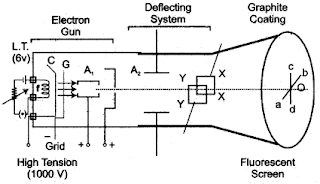Question 2: The figure (i) shows a radioactive source S placed in a thick walled lead container. The radiations given off pass through a magnetic field acting in direction perpendicular to the plane of paper inwards as shown by X. Copy the diagram and show the path of radiations. Explain why the source is kept in a thick-walled lead container.Answer 2: The figure (ii) shows the path of radiation in the magnetic field. The source is kept in a thick-walled lead container so that the it.
Radiations may not come out in the undesired direction otherwise they will harm the person working on.

Question 1: Answer the questions with respect to the following diagram:(i) Name the charged particles emitted from the heated filament? Name the phenomenon of emission of these particles.
(ii) A beam of such particles, after acceleration, is allowed to strike a metal plate C. Radiation B is given off. Name the radiation B.
(iii) Name the material of target C.
(iv) State two properties of radiation B different from those of visible light.
(v) State two factors on which the wavelength of radiation B depends.
(vi) State two uses of radiation B.
(vii)Why is it necessary to cool the tube?

Answer 1: (i) ‘Electrons’. The phenomenon of emission of these particles is called “thermionic emission”.
(ii) Radiation B is called “X-rays”.
(iii) The material of the target C is “tungsten.”
(iv) The properties of radiation B differ from those of visible light are:
(a) These radiations can penetrate through the substances which are opaque to visible light.
(b) They destroy the living cells.
(v) The wavelength of radiation B depends upon:
(a) Potential difference applied between cathode and anode.
(b) Material of the target.
(vi) Uses:
(a) In the field of medical science (X-rays photography used in surgery)
(b) In scientific work (to study crystal structure).
(vii) A large amount of heat is produced when the high kinetic energy electrons strike the target. Therefore it is necessary to cool the tube.

Question 2: An element P disintegrates by beta-emission and the new element suffers two further disintegrations, both by beta-emission, to form an element Q. Show that P and Q are isotopes.

Solution 2:The atomic numbers (Z) of P and Q are the same but their mass numbers [A and (A-4)] are different. So P and Q are isotopes.

Question 3: An element X has the symbol  84X202  . It emits an alpha-particle and then a beta-particle. The final nucleus is bYa . Find a and b.

Solution 3:Question 4: Complete the following fission reaction

Solution 4: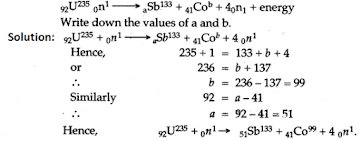### Long Numericals

Question 1: A certain nucleus X has a mass number 15 and atomic number 7. Find the number of neutrons. The nucleus X losses (i) one proton (ii) one β-particle, (iii) one α-particle, Express each change and write the symbol of the new nucleus in each case.

Solution 1: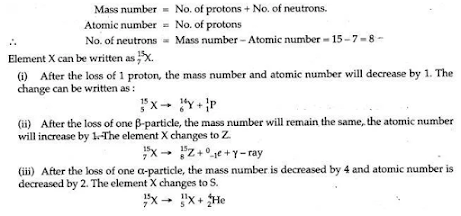Question 2: An element 92U238  losses one alpha particle, and then one β-particle and then again one β- particle to get the final nucleus bQa .
(i) Write down the values of a and b.
(ii) State the relationship between the nuclei P and Q.

Solution 2: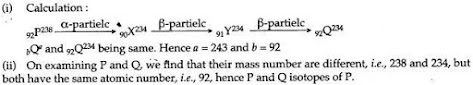Question 3: An isotope of uranium is 92Y238 ., i.e., it has mass number 235 and atomic number 92.
(i) Find the number of electrons in the neutral atom of this isotope.
(ii) Find the number of protons and neutrons in its nucleus.
(iii) Do all isotopes have the same number of neutrons?
(iv) What is the number of protons in 92U238 ?
(v) Mention the relationship between 92U235 and 92U238 .

Solution 3:

(i) Since it is a neutral atom, the number of proton will be equal to the number of electrons.

Number of protons = Atomic number = 92

∴ Number of electrons = 92

(ii) Atomic mass = Number of protons + Number of neutrons

Or, 235 = 92 + Number of neutrons

∴ Number of neutrons = 235 – 92 = 143

(iii) No. of isotopes of an element have the same atomic number but they differ in their atomic masses, and hence in such isotopes, the number of neutron will be different.

(iv) In 92U238, the atomic number is 92 and so the number of protons will also be 92.

(v) In 92U238 and 92U235, the difference is in their atomic masses but their atomic number is the same that is both have the same atomic number, 92. The element that differ in their atomic masses but have same atomic number are called isotopes, hence 92U238 and 92U235 are the isotopes of the element uranium.